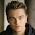## Friday, December 7, 2012

### Matrix trick and the ontology ball

Genifer's logic has the structure of a semi-ring obeying:

$\mbox{associative addition:} \quad (A + B) + C = A + (B + C)$
$\mbox{associative multiplication:} \quad (A \times B) \times C = A \times (B \times C)$
$\mbox{non-commutative multiplication:} \quad A \times B \neq B \times A$
$\mbox{distribution on the right}\quad (A + B) \times C = A \times C + B \times C$
$\mbox{commutative addition:} \quad A + B = B + A$
$\mbox{idempotent addition:} \quad A + A = A$

In short, multiplication is similar to "concatenation of letters" and addition is like "set union".  In programming (data-structure) terms, the algebra is like a set of strings composed of letters, and the letters are what I call concepts.

## Matrix trick

The matrix trick is simply to represent the elements of the algebra by matrices.  (This may be related to representation theory in abstract algebra).

What is a matrix?  A matrix is a linear transformation.  For example, this is an original image:

This is the image after a matrix multiplication:

In short, matrix multiplication is equivalent to a combination of rotation, reflection, and/or shear.  You can play with this web app to understand it intuitively.

So my trick is to represent each concept, say "love", by a matrix.  Then a sentence such as "John loves Mary" would be represented by the matrix product "john $\otimes$ loves $\otimes$ mary".

Each arrow represents a matrix multiplication.  The transformations need not occur on the same plane.

By embedding the matrices in a vector space (simply writing the matrix entries as a flat vector), I can "locate" any sentence in the vector space, or cognitive space.

Keep in mind that the rationale for using matrices is solely because $AB \neq BA$ in matrix multiplication, or:
John loves Mary $\neq$ Mary loves John.

## Ontology ball

This is another trick I invented:

It is just a representation of an ontology in space (as a ball).  To determine if $A \subset B$, we see if $A$ is located within the "light cone" of $B$.  Note that fuzzy $\in$ and $\subset$ can be represented by using the deviation from the central axis as a fuzzy measure.

I think this trick is important since it replaces the operation of unification (as in Prolog or first-order logic) with something spatial.  Traditionally, unification is done by comparing 2 logic terms syntactically, via a recursive-descent algorithm on their syntax trees.  This algorithm is discrete.  With this trick we can now perform the same operation using spatial techniques, which is potentially amenable to statistical learning.

Update:  Unfortunately I realized that the light-cone trick is not compatible with the matrix trick, as the matrix multiplication rotates the space which may destroy the $\in$ or $\subset$ relations (as they rely on straight rays projecting from the origin to infinity).  In fact, a matrix multiplication can be seen as a rotation of an angle $\theta$ which can expressed as a rational multiple of $\pi$ (or arbitrarily close if irrational).  Then we can always find a $k$ such that $A^k = Id$ where $Id$ is the identity.  In other words, we can find a product of any word, say "love", such that:
love $\circ$ love $\circ$ love ... = $Id$
which doesn't make sense.

I am still thinking of how to combine the 2 tricks.  If successful, it may enable us to perform logic inference in a spatial setting.#### 1 comment:

1.Thank you for this valuable information and in waiting for more from you. I regularly spend much time on lust looking for some worthy sites when I can find something to read.
Perceptual Wedge Hypothesis